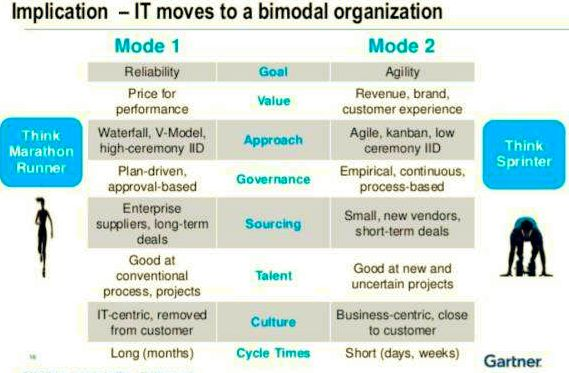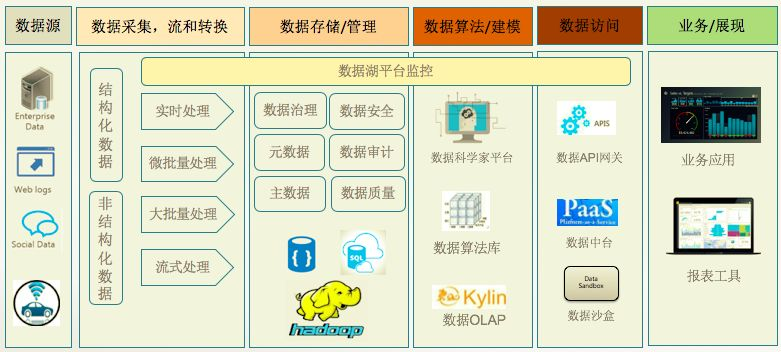## 技术干货 | 风口上的中台战略

2023年03月19日 14:00:55      来源：上海派拉软件股份有限公司 >> 进入该公司展台      阅读量：0

\r 人怕出名猪怕壮，阿里一出手，对手抖三抖…\r

\r

\r 言归正传，自从阿里提出“小前台，大中台”，逐渐演变成“中台战略”“中台思维”…\r

\r

\r
\r
\r

\r

\r 中台究竟是什么呢？
\r
\r

\r

\r \r

\r

\r 从字面上看，它应该是介于前台和后台之间的一层，称之为“中台”。我们通常理解的前台是业务系统，包括电商平台，客户服务系统之类的，后台是IT支撑，包括云计算平台，数据库，运维系统等等。\r

\r

\r

\r
\r

\r

\r

\r
\r

\r

\r

\r

\r

\r

\r

\r

\r

\r

\r

\r

\r\r

\r

\r 阿里巴巴中台架构的概况\r

\r

\r
\r

\r

\r 那么中台的本质是什么？\r

\r

\r

\r
\r
• \r 敏捷\r
• \r
\r

\r
\r
• \r 解耦\r
• \r
\r

\r
\r
• \r

\r  复用\r

\r
• \r
\r

一些公共的能力通过复用，大大提高了开发效率，避免了重复建设。同时使得数据和流程可以集中得以管理和优化。\r

\r

\r

\r那么中台应该包含些什么东西？\r

\r
\r

\r

\r 中台通常可以分为三个层面： 业务层面，数据层面和技术底层。\r

\r
\r
• \r 业务服务\r
• \r
\r

\r

\r
\r
• \r

\r  数据服务\r

\r
• \r
\r

\r

\r
\r
• \r 基础共享服务\r
• \r
\r

\r

\r

\r

\r

\r

\r\r

\r

\r
\r

\r
\r
• \r 建立合理的管理机制\r
• \r
\r

\r

\r

\r
\r

\r\r

\r

\r

\r

\r

\r

1、  搭建适合企业的应用架构\r

\r

\r

\r

Gartner将企业的两种IT称为双模IT，传统IT是模式1，互联网IT是模式2。\r

\r
\r
\r \r
\r

\r
\r

\r

\r\r

\r

\r
\r

\r

\r

\r

\r \r

\r

\r

\r
\r \r
\r\r

\r
\r

\r

\r\r

\r

\r
\r

\r

\r

\r

\r \r

\r

\r

2、提供基础共享服务\r

\r

\r\r

\r

3、数据中台\r

\r

\r

\r

\r

\r

\r\r

\r

4、业务中台 \r

\r

\r

\r

\r
\r

\r\r

\r

\r
\r

\r

\r

\r

5、中台相关基础架构\r

\r

\r\r

\r

\r
\r

\r

\r
\r
• \r

\r  DevOps：解决业务开发的敏捷性\r

\r
• \r
• \r

\r 微服务架构（MSA）：解决应用架构，平行开发，快速迭代\r

\r
• \r
• \r

\r 容器和编排：高度弹性，应对快速变化的业务压力\r

\r
• \r
• \r

\r 云：解决计算资源的统一调度\r

\r

\r
\r
\r

\r
• \r
\r\r

\r

\r
\r

\r

1.凡本网注明"来源：中非贸易网"的所有作品，版权均属于兴旺宝装备总站，转载请必须注明兴旺宝装备总站。违反者本网将追究相关法律责任。
2.企业发布的公司新闻、技术文章、资料下载等内容，如涉及侵权、违规遭投诉的，一律由发布企业自行承担责任，本网有权删除内容并追溯责任。
3.本网转载并注明自其它来源的作品，目的在于传递更多信息，并不代表本网赞同其观点或证实其内容的真实性，不承担此类作品侵权行为的直接责任及连带责任。其他媒体、网站或个人从本网转载时，必须保留本网注明的作品来源，并自负版权等法律责任。 4.如涉及作品内容、版权等问题，请在作品发表之日起一周内与本网联系。